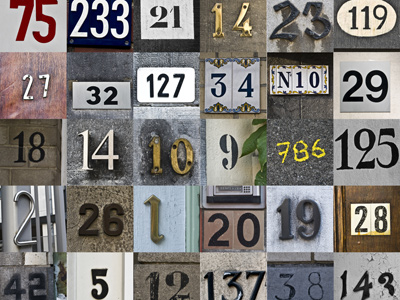These questions can seem like just a collection of random numbers!

# VR - Number Series Using Alternating Terms and Geometric Progression

As we found out in the previous article, Number Series are a common type of question in the Eleven Plus Verbal Reasoning exam. There are a few ways in which series of numbers can be constructed. In this lesson we look at alternating terms used in conjunction with geometric progression.

These can be very tricky to solve and are notorious for catching many candidates out! But, armed with the right techniques, they can be solved reasonably quickly.

Let’s look at some examples to show you what I mean.

Example Question One

Use the following series of numbers to determine what should come next.

 13 19 31 55 (?)

If the answer is not obvious, even when we’ve tried the techniques we’ve already learned, then there is another worth a go. Work out the difference between each adjoining number. If we did that then we would see the following:

 13 (6) 19 (12) 31 (24) 55

The series of numbers did not seem to have a great connection whereas, with the differences written in, we can see a clear progression. It’s effectively a two-part question – work out the differences and find the following number in that series, before adding it to the last number in the original sequence.

The series in brackets, 6, 12, 24 will be completed by the number 48 as it is a geometric progression, multiplying the first number by two to make the next. Therefore, the answer we need is 55 + 48 = 103.

Again, be wary of the mix of arithmetic and geometric progressions. A child who is only looking for the former could use the technique and spot the differences between the numbers, only to decide on the next number having a difference of 30 from the one before. This is the sort of thing the question setters look to exploit and there is almost guaranteed to be a 'wrong answer' that follows that logic.

Next, let’s revisit alternating terms:

Example Question Two

Use the following series of numbers to determine what should come next.

 15 6 21 8 27 (?)

There are two ways of approaching these questions. Firstly, follow the basic step suggested for example question one:

 15 (-9) 6 (+25) 21 (-13) 8 (+19) 27

This looks rather scary as there is clearly nothing simple about the progression. If you use logic you would assume that the next step would be to subtract a number from the 27 given that you subtract, add, subtract, add...

Given that the first two numbers to be subtracted are 9 and 13, the next should presumably be 17. (9 + 4 = 13, 13 + 4 = 17) The answer is therefore 10.

Now that was all very confusing for a child. Here’s where a good tutor would show you the best approach to solve these types of question.

## Technique Tip

When you see a series where the numbers do not all increase or decrease, try a different approach. The likelihood is that the first, third, fifth etc will be connected as one series and the second, fourth, sixth etc will be connected as another. Treat each separately and, instead of working out the difference between neighbouring numbers, work out the differences between alternate ones.

Using this advice, we can break down the bigger series into two smaller series:

 15 … 21 … 27

And

 6 … 8 …

The first series has numbers increasing by six each time. The second series involves the addition of two each step. We need to find the sixth number and that means we can ignore the first series. The sixth number is just the fourth number plus two. The answer is therefore 10 – and that is hopefully a lot easier than trawling through the alternative way!

If we remove half the question and are left with a simple arithmetic progression, we have a simpler task which will save your child a lot of time. Ensure they watch out for the numbers that increase and then decrease or vice-versa.

## Sample Tests

Number series using alternating terms combined with geometric progression are possibly the hardest question in the Eleven Plus Verbal Reasoning exam. But now you know how to tackle them, practising is the best way to build up your child’s confidence.

If you have already played the forty questions we have on Number Series, then you might like to revisit them, now that you have been armed with a more formidable weapon! For your convenience, here are some links to our quizzes:

Number Series 1

Number Series 2

Number Series 3

Number Series 4

There are still two more ways in which Number Series can be constructed. You might prefer to wait until after you’ve read the next two articles before you give them a go. See you there.